PT EN

## Tips and tricks

In this section you'll find a series of tutorials with step-by-step instructions showing how one can achieve somewhat complex constructions on TI-Nspire™. Some of the tutorials include exactly the same techiques emplyed in building the documents of the Programs section.

All tutorials are ranked according to difficulty and time needed to complete them.## Full turn angles

TI-Nspire's geometry tools allow us to build and measure angles, but only from 0 to 180 degrees (or 0 to π radians, depending on the definition of angular units. This tutorial describes how one can build and measure angles from 0 to 360 degrees and also how to use formulas that produce the same results, regardless of the angle measurement units chosen.

Time needed: 1h:30m
Difficulty: Intermediate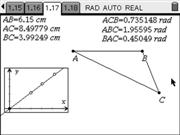## Cluttered screens

This tutorial show how one can use the Analytic window to display large quantities of information without cluttering the screen.

Time needed: 20 minutes.
Difficulty: Easy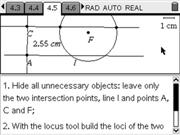## Conics

In this tutorial we explore the Locus tool so that we can build the three conics: ellipse, parabola and hyperbola. Throughout the tutorial you'll find step by step instructions.

Time needed: 40 minutes.
Difficulty: Easy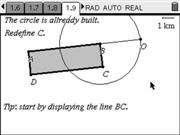## Geometry with constraints

This tutorial explains how one can use the Redefine tool to add restrictions to a geometrical construction and achieving a desired property. The problem studied is building a fixed perimeter rectangle, that can later be used on an activity about area maximization.

Time needed: 20 minutes.
Difficulty: Easy.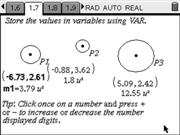## Connections between geometry and spreadsheet

This tutorial explores variables and how they can be used to extract information from a geometrical construction to another application and the other way around, linking geometrical properties to variables to get information from another application. The exercise given is building a construction to determine the position of the center of mass of a system of 3 particles in a way easy to generalize.

Time needed: 20 minutes.
Difficulty: Easy.## Random point generation

This tutorial explains how one can build a dynamic random point generator on the Geometry App. These types of generators can be used in a wide range of probabilistic simulators. Similar techniques were applied in the Monte Carlo simulation available on the Activities section of this site.

Time needed: 40 minutes.
Difficulty: Intermediate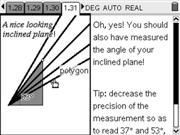## Angular restrictions

In this tutorial we explore the techniques that were used for building the Net force activity. We'll built a segment with angular restrictions so as to make only certain angles with a horizontal line and use this technique to build an inclined plane model that can be used in Physics activities.

Time needed: 1 hour.
Difficulty: Intermediate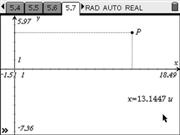## Objects locked in place

This tutorial explores techniques that will allow us to display information (being geometric objects, text messages or results of calculations) in a fixed position, regarless of window settings.

Time needed: 40 minutes.